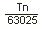Call 1-800-728-5168 | Email Us# Cylinder Formulas

For help with hydraulic calculations, we’ve provided a quick chart of the most popular hydraulic cylinder formulas such as cylinder area, velocity, capacity, flow rate, and more.  If you need more Hydraulic Pump information or are in search of a Hydraulic Repair Service, please contact us today!

## Hydraulic Cylinder Formulas

Cylinder Formulas For: Word Formula Letter Formula
Cylinder Area
In Square Inches
Area =x Radius2 (Inches)
Area =x Diameter2 (Inches)
A =r2
A =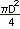or  A = .785D2
Cylinder Force
In Pounds, Push or Pull
Force = Pressure (psi) x Net Area (Square Inches) F = psi x A or F = PA
Cylinder Velocity or Speed
n Feet/Second
Velocity =V =or
V =Cylinder Volume Capacity
In Gallons of Fluid
Velocity =Velocity =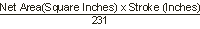V =V =/ = Length of Stroke
Cylinder Flow Rate
In Gallons Per Minute
Flow Rate =Q =or  Q = 3.117vA
Fluid Motor Torque
In Inch Pounds
Torque =Torque =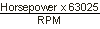Torque =T =or
T =T =T =or
T =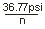Fluid Motor Torque/100 psi
In Inch Pounds
Torque/100 psi =T100psi =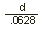Fluid Motor Speed
In Revolutions/Minute
Speed =n =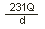Fluid Motor Power
In Horsepower Output
Horsepower =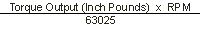HP =